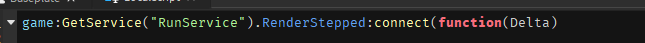# Custom Character Consistent Turn Speed

I currently have a custom movement system that includes the character turning in the direction they are moving with :lerp(). While the code works great when only using the WASD keys, there is one issue. Smaller movements such as by adjusting direction with the camera end up taking the same time as doing a 180, making it look very odd.

Here is what I have right now:

``````game:GetService("RunService").RenderStepped:connect(function()
local GoalDirection = MoveForce.PlaneVelocity --This is now the new direction
local SF = 1/FPS --typically 1/60

--Set to 15 times speed.
local Completion =  SF*15
if Completion >= 1 then
Completion = 1
end
local ReVector = Vector3.new(GoalDirection.X,0,GoalDirection.Y) --Convert Vect2 to Vect3

Root.CFrame = Root.CFrame:lerp(CFrame.new(Root.Position, Root.Position + ReVector),Completion)
end
end)
``````

How can I make the rotation speed consistent?

I dont quite understand the end goal of this code, but I would generally make something smoother by adding:“Delta” to my Heartbeat / Runservice
Its automatically passed to the function ( its Time between frames )

then if you needed the shorter rotation or movements to be faster then
I would get the magnitude of the distance for the players movement
and multiply the lerp value by some magnitude

I don’t know if you will like this or not, but

that is how I would do your lerp function ( it doesn’t add the magnitude modifier, but you can just add it into the clamp )

Sorry if it wasn’t clear what I said. My custom movement system uses two linear forces (one for “ground plane”, and the other for vertical movement. The goal is to make the player turn in the direction they move smoothly and consistently, like how the default movement system works. The direction the player needs to face is already obtained from the ground force.

Essentially, if you were turning 180 degrees, it should be twice as long as moving 90 degrees.

Anyways I tried getting the magnitude which kind of worked, but if you interrupted a rotation that was in proggress, the system wouldn’t account for where you currently were. This resulted in minor adjustments snapping you towards them. So I then lerped the third newest direction towards the second newest direction by the last ‘completion’ to find where the player is currently facing, then getting the magnitude. However, this apparently didn’t solve anything and the snapping bug persisted.

Here’s what the code looks like adjusting for magnitude:

``````CurrGoalDirection = Vector2.new()
LastStartVelocity = Vector2.new()
Completion = 0
Scale= 0

game:GetService("RunService").RenderStepped:connect(function(Delta)
if MoveForce.PlaneVelocity ~= Vector2.new(0,0) then  --make sure we are moving
if CurrGoalDirection ~= MoveForce.PlaneVelocity then --New force
--[[ Magnitudes per angle
Angle of 45: 18-19,
Angle of 90: 35,
Angle of 180: 48-50
]]
local CurrentForce = LastStartVelocity:Lerp(CurrGoalDirection,Completion)
Scale = (MoveForce.PlaneVelocity- CurrentForce).Magnitude
LastStartVelocity = CurrGoalDirection
CurrGoalDirection = MoveForce.PlaneVelocity --This is now the new direction
end
local Booster = 200/math.ceil(Scale + 0.5) -- 200 is just a test number.

--Multiply frames passed by total frames, then set to 3 times speed.
Completion = math.clamp(Delta*Booster,0,1)

local ReVector = Vector3.new(CurrGoalDirection.X,0,CurrGoalDirection.Y) --Convert Vect2 to Vect3

Root.CFrame = Root.CFrame:lerp(CFrame.new(Root.Position, Root.Position + ReVector),Completion)
end
end)``````

The issue here is that Completion is always set to a constant value of 15 * SF (e.g. 15/60). This means that regardless of the direction or magnitude of the goal direction, the character will always turn at the same speed.

To make the rotation speed consistent, you will need to adjust the Completion variable based on the magnitude of the goal direction. You can do this by calculating the magnitude of the goal direction, then dividing that by a desired maximum speed. This will result in a value between 0 and 1, which can then be used for the Completion variable.

Here is an example of what the updated code could look like:

``````game:GetService("RunService").RenderStepped:connect(function()
local GoalDirection = MoveForce.PlaneVelocity --This is now the new direction
local SF = 1/FPS --typically 1/60

--Calculate magnitude of GoalDirection
local GoalDirectionMagnitude = GoalDirection.magnitude

--If magnitude is greater than 1, set it to 1
if GoalDirectionMagnitude > 1 then
GoalDirectionMagnitude = 1
end

--Set to 15 times speed
local Completion = GoalDirectionMagnitude * SF * 15
if Completion >= 1 then
Completion = 1
end
local ReVector = Vector3.new(GoalDirection.X,0,GoalDirection.Y) --Convert Vect2 to Vect3

Root.CFrame = Root.CFrame:lerp(CFrame.new(Root.Position, Root.Position + ReVector),Completion)
end
end)``````

I’ve already acomplished something similar using align orientation. You can check it out here under the AutoRotate module component:

Thanks, seems to be consistent now.

This topic was automatically closed 14 days after the last reply. New replies are no longer allowed.